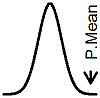P.Mean: Power calculations for comparison of Poisson counts across two groups (created 2010-01-11).

Suppose you want to compare Poisson count variables across two groups. How much data would you need to collect? It's a tricky question and there are several approaches that you can consider.

Here's the basic setup. You watch a group of N1 patients in group 1 for T1 patient years and record X1, the number of events that occur in this group. At the same time, you watch a group of N2 patients in group 2 for T2 patient years and record X2, the number of events that occur in this group. A reasonable assumption is that the two counts follow a Poisson distribution.You wish to test the hypothesis that the events occur at the same rate:How much data would you need in order to insure that this hypothesis test has sufficient power? It's a tricky question, and the answer will be defined in terms of the total number of observed events (X1 + X2). With that data, you can calculate the the number of patients years of followup (T1 + T2). If the average followup time per patient is known, then you can also calculate the total number of patients (N1 + N2).

Power for testing a rate ratio. The rate X1/T1 is a reasonable estimate of λ1 and the rate X2/T2 is a reasonable estimate of λ2. The ratio of these two rates,is going to be close to R =  λ1 /  λ2, which will be equal to 1 when λ1 =  λ2.

As long as the counts are reasonably large, the log of the rate ratio is approximately normally distributed. The mean will beand the standard deviation will beUnder the null hypothesis, of course, the mean will be 0.

Select X1 and X2 so that you have adequate power for the test of the hypothesis that the rate ratio equals 1.

We would reject the null hypothesis of a rate ratio of 1 if the log of observed rate ratio is larger thanor smaller thanWe can ignore the second limit in this situation. If the true rate ratio is  λ1 /  λ2, then the power of the test is computed asStandardize the left hand side to get the following equation.This is equivalent toorThe right hand side of the equation is the harmonic mean of the number of events in the two groups.

Here's an example.

The control group in a study is assumed to have a Poisson rate of 0.26 events per patient year. We will follow patients in the two groups for the same amount of time, and we expect, based on previous studies that the average patient will have 1.7 years of follow-up time. We want to sample a sufficient number of patients so that if the treatment group has a rate 1.5 times as large (0.39 events per patient year), we would have 80% power for detecting this difference. Assume a two sided test with an alpha level of 0.05.

In this example,Since the number of events in the second group should be 1.5 times the number of events in the first group, trial and error will produce event counts of 78 and 117. If you were mathematically savvy, you would know to divide and multiply by the square root of 1.5 to get these numbers, but most of us are happy using trial and error.

A rate of 0.26 events per year would require 78 / 0.26 = 300 patient years of data. Each patient contributed 1.7 patient years of data in the previous study, so this would require 300 / 1.7 = 177 patients in the first group. If you repeat these calculations for the second group, you will get 117 / 0.39 = 300 patient years of follow-up and 300 / 1.7 = 177 patients for the second group as well.

Power for a simple binomial test. If we observe observe X1 and X2 events, then the distribution of X1 and X2 given the sum X1 + X2 is binomial. The probability of success for the binomial random variable isand the number of trials isUnder the null hypothesis that the two rates are the same, this probability simplifies toSelect a total number of events across both groups, N, so that the power of the test ofunder the alternative p=pA is sufficiently large. The formula for the total number of events across both groups is well known:Example: Assume again that the rate in the two groups are 0.26 and 0.39. If you want the same number of patient years in the first and second groups, then P0 = 0.5 and PA = 0.26 / (0.26 +0.39) = 0.4.You want 40% of these events in the first group (78) and 60% in the second group (117). As above, this means 300 patient years in each group and 177 patients in each group.This work is licensed under a Creative Commons Attribution 3.0 United States License. This page was written by Steve Simon and was last modified on 2017-06-15. Need more information? I have a page with general help resources. You can also browse for pages similar to this one at Category: Poisson regression or Category: Sample size justification.